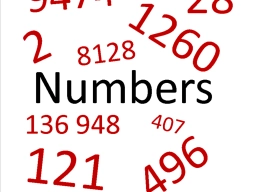# Determine 80714

Three different numbers are given. The average of the average of two smaller numbers and the average of the two larger numbers is equal to the average of all three numbers. The average of the smallest and largest number is 2022. Determine the sum of the three given numbers.

s =  6066

### Step-by-step explanation:Did you find an error or inaccuracy? Feel free to write us. Thank you!

Tips for related online calculators
Looking for help with calculating arithmetic mean?
Looking for a statistical calculator?
Do you have a linear equation or system of equations and looking for its solution? Or do you have a quadratic equation?

#### Grade of the word problem:

We encourage you to watch this tutorial video on this math problem: